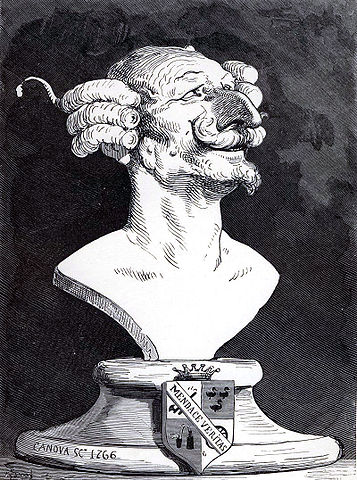# Münchausen numbersBaron Münchausen

The number 3435 has the following curious property:

3435 = 33 + 44 + 33 + 55.

It is called a Münchausen number, an allusion to fictional Baron Münchausen. When each digit is raised to its own power and summed, you get the original number back. The only other Münchausen number is 1.

At least in base 10. You could look at Münchausen numbers in other bases. If you write out a number n in base b, raise each of its “digits” to its own power, take the sum, and get n back, you have a Münchausen number in base b. For example 28 is a Münchausen number in base 9 because

28ten = 31nine = 33 + 11

Daan van Berkel proved that there are only finitely many Münchausen in any given base. In fact, he shows that a Münchausen number in base b cannot be greater than 2bb, and so you could do a brute-force search to find all the Münchausen numbers in any base.

The upper bound 2bb grows very quickly with b and so brute force becomes impractical for large b. If you wanted to find all the hexadecimal Münchausen numbers you’d have to search 2*1616 = 36,893,488,147,419,103,232 numbers. How could you do this more efficiently?

## 4 thoughts on “Münchausen numbers”

1. There are far fewer sums-of-squared-digits up to a given limit than there are numbers up to the same limit, because addition is commutative. So for starters: instead of visiting every number up to the limit and seeing whether it has the Münchausen property, visit only the numbers that have their digits in non-increasing order and see whether its sum-of-squared-digits is any *permutation* of the number. If so, then the sum so found is a Münchausen number.

A further idea, not completely thought out: for a given number, iterate taking the sum of squared digits (as in oeis.org/A000216). Along the way, mark every number you see, and every permutation of every number you see, as visited. Iterate until you reach a previously visited number, or go above the 2b^b limit. If you end by finding a period-1 cycle, you have a Münchausen number. Continue with the next un-visited number up to the limit.

I think this can be accomplished using O(b log b) bits of storage: 2b^b, written in base b, will be b+1 digits long (for b>2). We can represent a number, up to permutation, as a histogram of digits. The histogram will have b bins, and each value can hold a value from 0 to b+1, which takes O(log b) bits.

2. Whoops, went off the rails in the last paragraph — that doesn’t make for the *total* storage. It’s late and I need sleep, so I won’t try to fix it right now, just ignore it.

3. made a Python version based on the idea of combinations+permutations of the digits. It handles both 0^0=1 and 0^0=0 conventions (which allows 438579088 as Munchhausen number in base 10)

Code and first results is here : https://gist.github.com/goulu/5121c161d224229b76a38485c4122794

Still processing for base 15 and 16…